# 1.学习总结

## 1.1查找的思维导图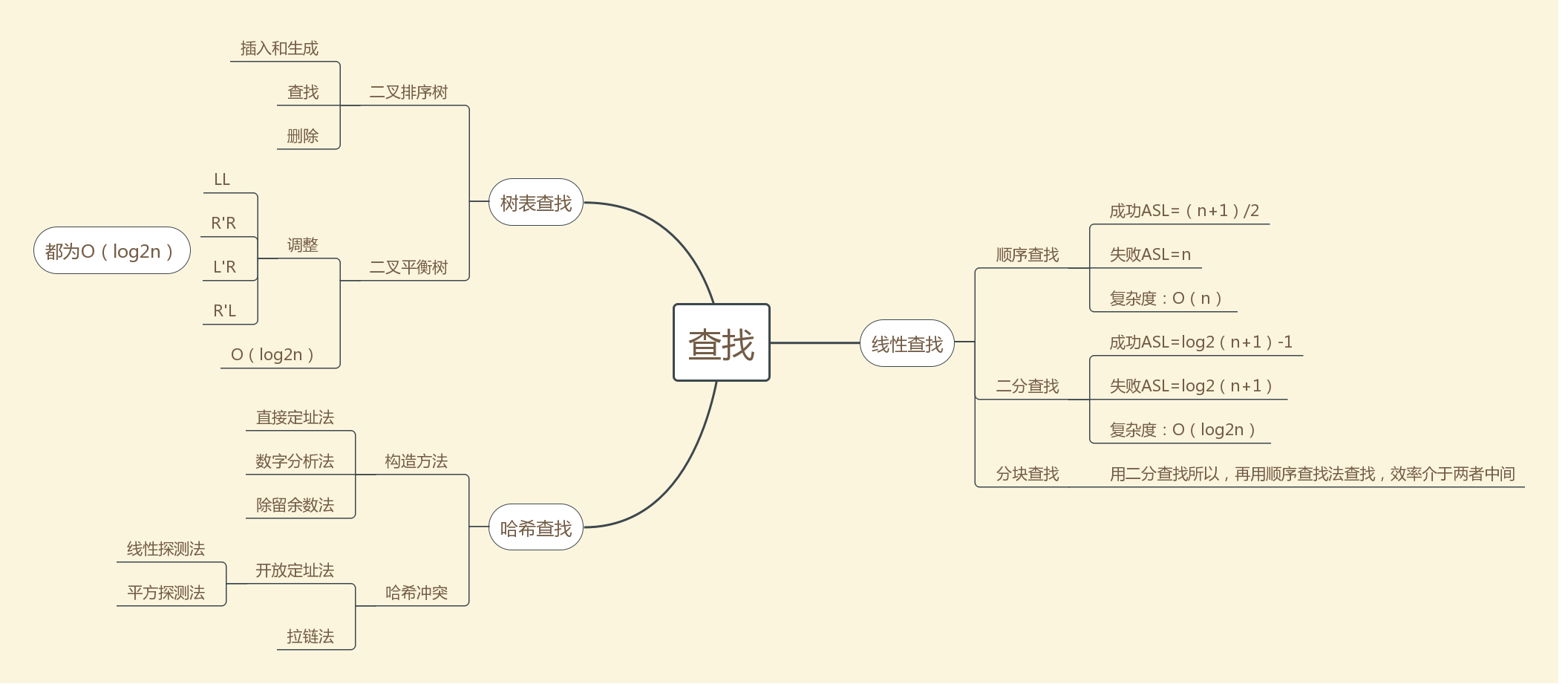# 2.PTA实验作业

### 2.2 设计思路

如果是空树
返回 true

返回 true

返回 false

返回 false



### 2.3 代码截图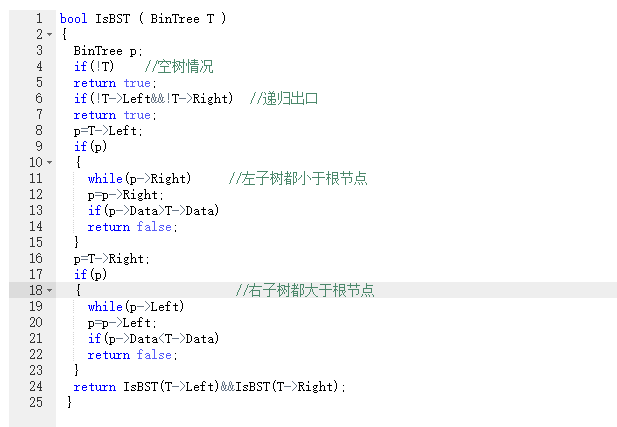### 2.4 PTA提交列表说明。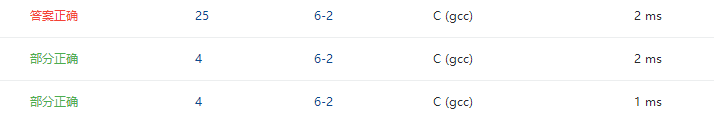### 2.2 设计思路

Find 函数
{
如果T为空
返回0  递归出口
如果a大于T的值
就往T的右树寻找
如果a小于T的值
就往T的左树寻找
如果找到
就返回1
}

LCA 函数
{
如果T为空
返回error
如果树中没有u和v的值
返回error
如果u和v一个比T的值大一个小
T就是他们的共同最近祖先
如果u和v有一个跟T的值相同
T就是他们的共同最近祖先
如果u和v都小于T的值
就往T的左树寻找
如果u和v的值都大于T的值
就往T的右树寻找
}


### 2.3 代码截图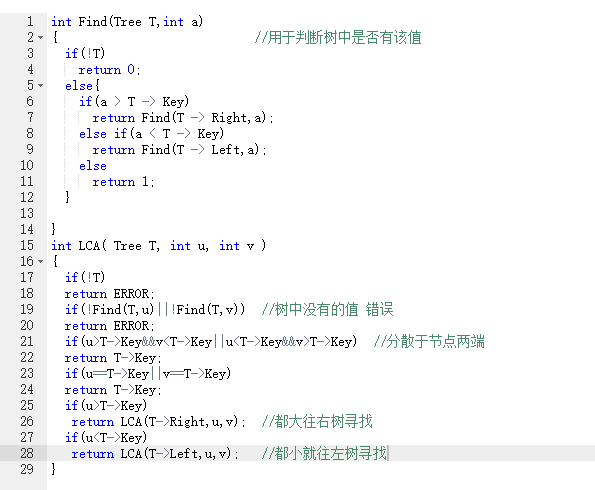### 2.4 PTA提交列表说明。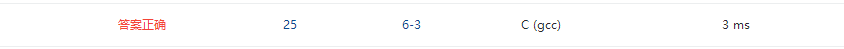### 2.2 设计思路

输入的字符为'N'时
{    判断里面是否有该账号记录
若没有{  输出ok 并把数据输入}
若有{ 输出Exist}   }

{      判断里面是否有该账号的记录
若没有{ 输出 Exist}
若有{  进一步判断密码是否正确 } }


### 2.3 代码截图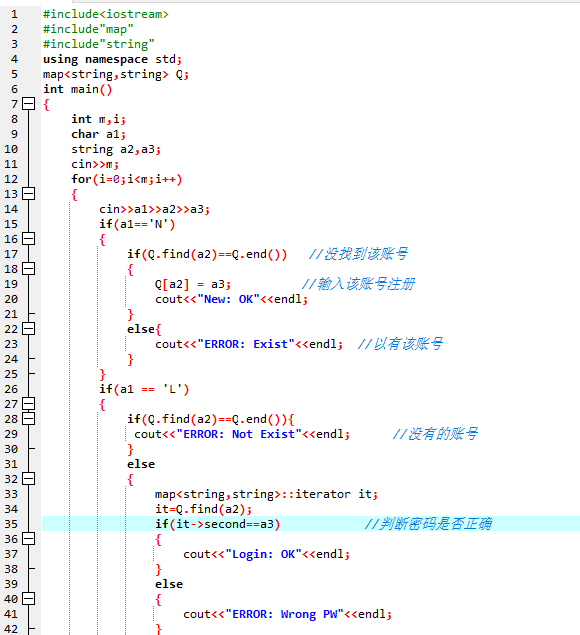### 2.4 PTA提交列表说明。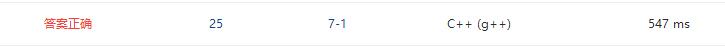# 3.截图本周题目集的PTA最后排名

### 3.1 PTA排名：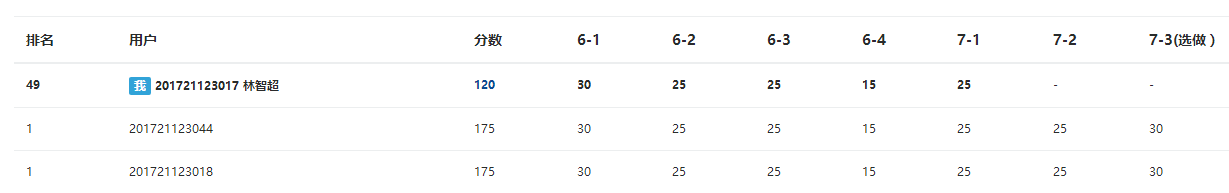## 4. 阅读代码

class Solution {
public:

int calculate(int n)
{
int squared_sum = 0;
while(n)
{
int tmp = n%10;
squared_sum += tmp * tmp;
n/=10;
}
return squared_sum;
}
bool isHappy(int n)
{
bool isHappyNum = false;
unordered_set<int> mySet;
while(true)
{
//the final num is 1
if(n == 1)
{
isHappyNum = true;
break;
}

//a number appears more than once,indicating that falling into a loop with out 1
if(mySet.find(n) != mySet.end())
{
isHappyNum = false;
break;
}

mySet.insert(n);
//calculate the squared sum of every single digit in n
n = calculate(n);
}

return isHappyNum;

}
};


posted @ 2018-05-26 21:56  圣多美  阅读(199)  评论(1编辑  收藏  举报